### Home > A2C > Chapter 6 > Lesson 6.2.1 > Problem6-65

6-65.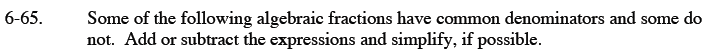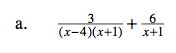$\frac{3}{(x-4)(x+1)}+\frac{6}{x+1}\cdot \frac{x-4}{x-4}$

$\frac{3+6(x-4)}{(x-4)(x+1)}$

$\frac{6x-21}{(x-4)(x+1)}$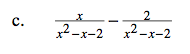Try factoring after subtracting.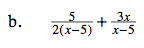See part (a).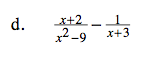Factor to find the common denominator, then use the strategy from part (a) to simplify.

$\frac{5}{x^2-9}$

$\text{If your answer was }-\frac{1}{x²-9},$

check the step where you subtracted.

−(x − 3) = x + 3